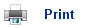# Buffer Solutions

• A buffer is a solution in which the pH resists change when a small amount of strong acid or base is added.
• Buffers consist of either a weak acid and salt (acid buffer), or a weak base and salt (a base buffer).
• Acid buffers maintain pHĺs on the acid side of neutrality (<7); base buffers maintain the pH on the basic side of neutrality.
• The action of buffer solutions can be understood by looking at the dynamic equilibrium that occurs between a weak acid and its conjugate base in water:

HA(aq) + H2O(l)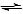H3O+(aq) + A-(aq)

• If a strong acid is added to the solution, the position of equilibrium will shift to oppose the change; the position of equilibrium will move to the left. The newly formed H3O+ ions will transfer protons to the A- ions forming the weak acid and H2O molecules.
• Because the added H+ ions are removed by the solution by the A- ions, the pH of the solution remains almost unchanged; the A- ions have acted as a sink for the H+ ions.
• If a strong base is added to the solution, then the incoming OH- ions will remove protons from the HA molecules to form H2O and A- ions. In this case, the HA has acted as a source of H+ ions; consequently the pH of the solution remains unchanged.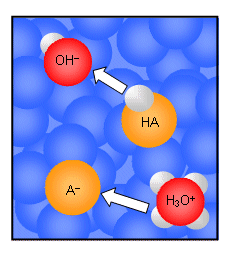• The same occurs with base buffers; if strong acid is added, then the basic salt, NH3 for example, will accept protons from the acid becoming NH4+.
• If strong base is added, then the base, NH4+, will donate protons to it, becoming NH3.
• A number of assumptions must be made when considering bases:
1. All the A- ions come from the salt. The weak acid, HA, supplies very few A- ions to the solution in comparison to the fully ionised salt.
2. Almost all of the HA molecules put into the buffer remain unchanged. If the HA molecules dissociate then the pH will change; however, very few of them do as the position of equilibrium for the dissociation of the weak acid is far to the left, due to presence of H+ and A- ions.

## Calculations involving buffers

• In order to perform calculations on buffer solutions, all is needed is the Ka expression for the relevant weak acid: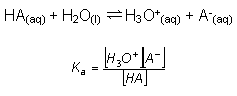• Rearranging this gives: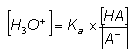• The value of the [H3O+], and therefore the pH of the solution depends on:
1. The value of Ka
This provides the course tuning for the buffer solution; Ka values are usually within the pH range of 4-10. Selection of a particular weak acid determined the region of the pH range the buffer is in.
2. The ratio of [salt]:[acid]
The provides the fine tuning for the pH of the buffer. Changing the ration from around 3:1 to 1:3 changes the [H+] by a factor of approximately 9, and therefore changes the value of the pH by 1 unit. The ratio should not be too far outside this range, otherwise there will not be enough acid or salt.
• The buffer is not affected by dilution, as when water is added, both the concentration of the weak acid and the salt change by the same amount; therefore their ratio of concentrations remains unchanged.

## Example Calculation

• A buffer solution consisting of 0.040 mol dm-3 CH3COO-Na+(aq) and 0.08 mol dm-3 CH3COOH(aq) is prepared at 1 atm, 298 K. Calculate the pH of the buffer solution.
• We first need to begin by identifying the weak acid and its conjugate base; we then need to write the equilibrium between the two:

CH3COOH(aq)CH3COO-(aq) + H+(aq)

• This is a reaction at dynamic equilibrium and so an Ka expression can be written: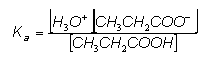• We can rearrange the equation to give:• From the data book, the Ka value for ethanoic acid is 1.78 x 10-5, so: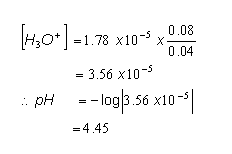## Use of Buffers

• Buffer solutions are present in many living systems, where reactions must take place under narrow pH ranges; for example our blood is buffered to pH 7.35-7.45, our saliva to pH 6.4-6.8 and our stomach juices to pH 1.6-1.8.
• Carbonic acid in the oceans acts as a buffer solution:

In low pHĺs CO32- ômops upö the excess H+ ions:

CO32-(aq) + H+ (aq)HCO3- (aq)

At high pHĺs the reverse of the reaction occurs:

HCO3-(aq)CO32-(aq) + H+(aq)

• Many chemical processes also require buffer solutions; dyeing fabrics can be ineffective at the wrong pH values.

## Useful books for revision

Revise A2 Chemistry for Salters (OCR A Level Chemistry B)Salters (OCR) Revise A2 ChemistryHome